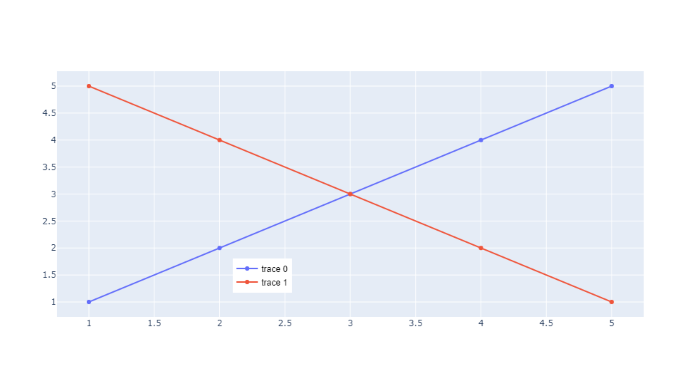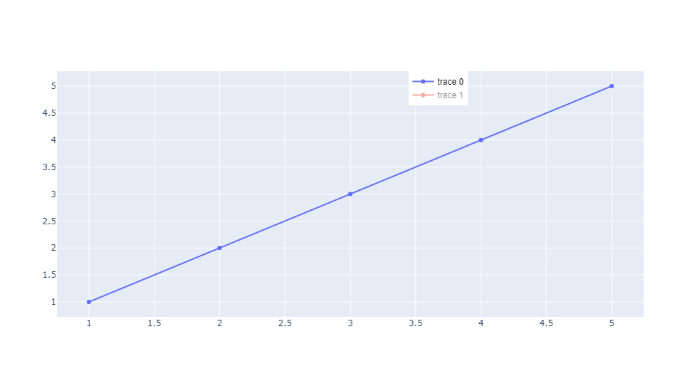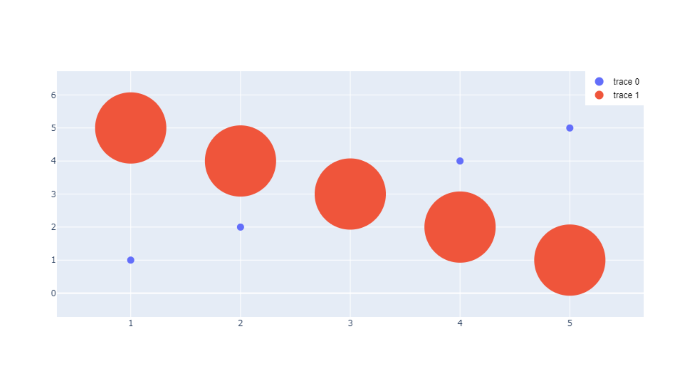Open in App
Not now

# How to position legends inside a plot in Plotly-Python?

• Last Updated : 28 Nov, 2021

In this article, we will learn How to hide legend with Plotly Express and Plotly. A legend is an area describing the elements of the graph. In the plotly legend is used to Place a legend on the axes.

Example 1:

In this example, we are positioning legends inside a plot with the help of method fig.update_layout(), by passing the position as x=0.3 and y=0.1.

## Python3

 `# importing packages ` `import` `plotly.graph_objects as go ` ` `  `# using medals_wide dataset ` `fig ``=` `go.Figure() ` ` `  `# plotting the scatter chart ` `fig.add_trace(go.Scatter( ` `    ``x``=``[``1``, ``2``, ``3``, ``4``, ``5``], ` `    ``y``=``[``1``, ``2``, ``3``, ``4``, ``5``], ` `)) ` ` `  `# plotting the scatter chart ` `fig.add_trace(go.Scatter( ` `    ``x``=``[``1``, ``2``, ``3``, ``4``, ``5``], ` `    ``y``=``[``5``, ``4``, ``3``, ``2``, ``1``], ` `)) ` ` `  `#  position legends inside a plot ` `fig.update_layout( ` `    ``legend``=``dict``( ` `        ``x``=``0.3``,  ``# value must be between 0 to 1. ` `        ``y``=``.``1``,   ``# value must be between 0 to 1. ` `        ``traceorder``=``"normal"``, ` `        ``font``=``dict``( ` `            ``family``=``"sans-serif"``, ` `            ``size``=``12``, ` `            ``color``=``"black"` `        ``), ` `    ``) ` `) ` ` `  `fig.show()`

Output:Example 2:

In this example, we are positioning legends inside a plot with the help of method fig.update_layout(), by passing the position as x=0.6 and y=1.

## Python3

 `# importing packages ` `import` `plotly.graph_objects as go ` ` `  `# using medals_wide dataset ` `fig ``=` `go.Figure() ` ` `  `# plotting the scatter chart ` `fig.add_trace(go.Scatter( ` `    ``x``=``[``1``, ``2``, ``3``, ``4``, ``5``], ` `    ``y``=``[``1``, ``2``, ``3``, ``4``, ``5``], ` `)) ` ` `  `# plotting the scatter chart ` `fig.add_trace(go.Scatter( ` `    ``x``=``[``1``, ``2``, ``3``, ``4``, ``5``], ` `    ``y``=``[``5``, ``4``, ``3``, ``2``, ``1``], ` `    ``visible``=``'legendonly'` `)) ` ` `  `# position legends inside a plot ` `fig.update_layout( ` `    ``legend``=``dict``( ` `        ``x``=``0.6``,  ``# value must be between 0 to 1. ` `        ``y``=``1``,  ``# value must be between 0 to 1. ` `        ``traceorder``=``"normal"``, ` `        ``font``=``dict``( ` `            ``family``=``"sans-serif"``, ` `            ``size``=``12``, ` `            ``color``=``"black"` `        ``), ` `    ``) ` `) ` ` `  `fig.show()`

Output:Example 3:

In this example, we are positioning legends inside a plot with the help of method fig.update_layout(), by passing the position as x=0.9 and y=1.

## Python3

 `import` `plotly.graph_objects as go ` ` `  `fig ``=` `go.Figure() ` ` `  `fig.add_trace(go.Scatter( ` `    ``x``=``[``1``, ``2``, ``3``, ``4``, ``5``], ` `    ``y``=``[``1``, ``2``, ``3``, ``4``, ``5``], ` `    ``mode``=``'markers'``, ` `    ``marker``=``{``'size'``: ``10``} ` `)) ` ` `  `# plotting the scatter chart ` `fig.add_trace(go.Scatter( ` `    ``x``=``[``1``, ``2``, ``3``, ``4``, ``5``], ` `    ``y``=``[``5``, ``4``, ``3``, ``2``, ``1``], ` `    ``mode``=``'markers'``, ` `    ``marker``=``{``'size'``: ``100``} ` `)) ` ` `  `# position legends inside a plot ` `fig.update_layout( ` `    ``legend``=``dict``( ` `        ``x``=``.``9``,  ``# value must be between 0 to 1. ` `        ``y``=``1``,   ``# value must be between 0 to 1. ` `        ``traceorder``=``"normal"``, ` `        ``font``=``dict``( ` `            ``family``=``"sans-serif"``, ` `            ``size``=``12``, ` `            ``color``=``"black"` `        ``), ` `    ``) ` `) ` ` `  `fig.update_layout(legend``=``{``'itemsizing'``: ``'constant'``}) ` ` `  `fig.show() `

Output:My Personal Notes arrow_drop_up
Related Articles